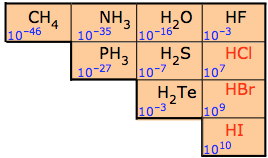# How does bond polarity affect the strength of an acid?

Mar 18, 2014

Increasing the polarity of an $\text{H-X}$ bond increases the strength of the acid.

#### Explanation:

When shared electrons in an $\text{H-X}$ bond spend most of their time close to $\text{X}$ atom, the bond becomes polar.

It also becomes weaker.

It is easier for the proton to leave the molecule, so the compound becomes more acidic.

The electronegativity of elements in the same row of the Periodic Table increases from left to right.

Thus, the polarity and the acidity of the $\text{H-X}$ bond increase in the order

$\text{H-C < H-N < H-O < H-F}$ and
$\text{H-Si < H-P < H-S < H-Cl}$

The chart below shows how acid strength increases from left to right across a row and from bottom to top in the Periodic Table